Essential Concept 3: Analysis of Variance (ANOVA) | IFT World
101 Concepts for the Level I Exam

# Essential Concept 3: Analysis of Variance (ANOVA)Analysis of variance is a statistical procedure for dividing the variability of a variable into components that can be attributed to different sources. We use ANOVA to determine the usefulness of the independent variable or variables in explaining variation in the dependent variable.
ANOVA table

 Source of variation Degrees of freedom Sum of squares Mean sum of squares Regression (explained variation) k RSS MSR=RSS/k Error (unexplained variation) n – 2 SSE MSE=SSE/(n-k-1) Total variation n – 1 SST

n represents the number of observations and k represents the number of independent variables. With one independent variable, k = 1. Hence, MSR = RSS and MSE = SSE / (n – 2).
Information from the ANOVA table can be used to compute:

• item Standard error of estimate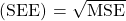• item Coefficient of determination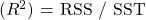The F-statistic tests whether all the slope coefficients in a linear regression are equal to 0. In a regression with one independent variable, this is a test of the null hypothesis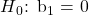against the alternative hypothesis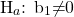. It measures how well the regression equation explains the variation in the dependent variable.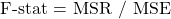** IFT Website Un-available on Monday 2-October-2023, 0700-1200 GMT **
This is default text for notification bar